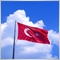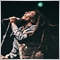# Indicators with alerts/signal - page 157934

`Thanks William but that's not what I indicated, it's similar but not the same. It does not use Bollinger bands but standard deviations. I have the indicator on my MT4 but I wanted the same on the MT5. If I provide the code, can someone translate it for the Metatrader 5?`34

```//+------------------------------------------------------------------+
//|                                       Market_Statistics_v4_2.mq4 |
//|                                             akifusenet@gmail.com |
//| Volume histogram implementation is based on Vadim Shumilov       |
//| (DrShumiloff)'s VolumesHist2.3 indicator. Other concepts are     |
//|                                                                  |
//| History:                                                         |
//| 17.01.2010: v4.2=>Small Bugfix [zero division possibility ]      |
//|                 =>When time period change call deinit and init   |
//| 09.09.2009: v4.1=>Small bugfix [delete startDate correct prefix] |
//| 09.09.2009: v4=>Instead of fixed start date now we have a        |
//|               =>relative startdate like 2 days back at 22:00     |
//| 02.09.2009: v3=>Calculation done only at the start of a new bar  |
//|               =>implementation is corrected                      |
//|               =>histogram defaulted 50 bar sd3show made true     |
//| 10.08.2009: v2=>End date added.SD2Show defaulted to true         |
//|               =>Show/disable options added for every line        |
//| 06.08.2009: v1=>Initial release                                  |
//|                                                                  |
//+------------------------------------------------------------------+

#property indicator_chart_window

#property indicator_buffers 8

#property indicator_color1 Orange      //PVP
#property indicator_width1 2

#property indicator_color2 Aqua        //VWAP
#property indicator_width2 2

#property indicator_color3 Yellow       //SD1Pos
#property indicator_width3 1
#property indicator_style3 2

#property indicator_color4 White         //SD1Neg
#property indicator_width4 1
#property indicator_style4 2

#property indicator_color5 Yellow//SD2Pos
#property indicator_width5 1
#property indicator_style5 2

#property indicator_color6 White     //SD2Neg
#property indicator_width6 1
#property indicator_style6 2

#property indicator_color7 Yellow   //SD2Pos
#property indicator_width7 1
#property indicator_style7 2

#property indicator_color8 White   //SD2Neg
#property indicator_width8 1
#property indicator_style8 2

//---- input parameters
extern string  label="Market_Statistics_1";
extern int     daysBack=1;
extern int     startHour=22;
extern int     startMinute=00;
extern int     HistogramAmplitude = 50;
extern bool    Show_SD1 = true;
extern bool    Show_SD2 = true;
extern bool    Show_SD3 = true;
extern bool    Show_Histogram = true;
extern bool    Show_PVP = true;
extern bool    Show_VWAP = true;

//---- buffers
double PVP[];
double VWAP[];
double SD1Pos[];
double SD1Neg[];
double SD2Pos[];
double SD2Neg[];
double SD3Pos[];
double SD3Neg[];

double Hist[]; // drawn specifically

int      period         =-2;
double   OpenTime       = 0;  // To check if we have a new bar
int      Bars_Back      = 0;  // Shows the starting bar for given date
int      items;               // numbers of items inside volume histogram
double   SD;                  // standart deviation
string   OBJECT_PREFIX;

// Finds the bar number for the given date
int FindStartIndex()
{
int dayofweektoday= TimeDayOfWeek(Time);
int days=0;

for (int i=1; i<=Bars; i++) {

if ((TimeDayOfWeek(Time[i])!=dayofweektoday)||(daysBack==0)) {
days++;
dayofweektoday=TimeDayOfWeek(Time[i]);

if ((daysBack==days)||(daysBack==0))
{
while ((TimeHour(Time[i])>startHour)||(TimeMinute(Time[i])>startMinute))
{
i++;
}

return (i);
}
}
}

return( 0);
}

int init()
{
OBJECT_PREFIX = label+"_VolumeHistogram_" + DoubleToStr(Time[FindStartIndex()],0)+"_" ;

//---- indicators
IndicatorBuffers(8);

if (Show_PVP==true) SetIndexStyle(0,DRAW_LINE);
else  SetIndexStyle(0,DRAW_NONE);
SetIndexLabel(0,"PVP");
SetIndexBuffer(0,PVP);

if (Show_VWAP==true) SetIndexStyle(1,DRAW_LINE);
else  SetIndexStyle(1,DRAW_NONE);
SetIndexLabel(1,"VWAP");
SetIndexBuffer(1,VWAP);

if (Show_SD1==true) SetIndexStyle(2,DRAW_LINE);
else  SetIndexStyle(2,DRAW_NONE);
SetIndexLabel(2,"SD1Pos");
SetIndexBuffer(2,SD1Pos);

if (Show_SD1==true) SetIndexStyle(3,DRAW_LINE);
else  SetIndexStyle(3,DRAW_NONE);
SetIndexLabel(3,"SD1Neg");
SetIndexBuffer(3,SD1Neg);

if (Show_SD2==true) SetIndexStyle(4,DRAW_LINE);
else  SetIndexStyle(4,DRAW_NONE);
SetIndexLabel(4,"SD2Pos");
SetIndexBuffer(4,SD2Pos);

if (Show_SD2==true) SetIndexStyle(5,DRAW_LINE);
else  SetIndexStyle(5,DRAW_NONE);
SetIndexLabel(5,"SD2Neg");
SetIndexBuffer(5,SD2Neg);

if (Show_SD3==true) SetIndexStyle(6,DRAW_LINE);
else  SetIndexStyle(6,DRAW_NONE);
SetIndexLabel(6,"SD3Pos");
SetIndexBuffer(6,SD3Pos);

if (Show_SD3==true) SetIndexStyle(7,DRAW_LINE);
else  SetIndexStyle(7,DRAW_NONE);
SetIndexLabel(7,"SD3Neg");
SetIndexBuffer(7,SD3Neg);

string short_name="Market_Statistics";
IndicatorShortName(short_name);

return(0);
}

// Delete all objects with given prefix
void ObDeleteObjectsByPrefix(string Prefix)
{
int L = StringLen(Prefix);
int i = 0;
while(i < ObjectsTotal())
{
string ObjName = ObjectName(i);
if(StringSubstr(ObjName, 0, L) != Prefix)
{
i++;
continue;
}
ObjectDelete(ObjName);
}
}

int start()
{
double TotalVolume=0;
double TotalPV=0;
int n;

if (period!=Period()&&(period!=-2))
{
Print("Period has been changed. Recalculation needed");
deinit();
init();
}
period=Period();

if (OpenTime != Time)
{
Bars_Back=FindStartIndex();
if (Bars_Back!=0)
{
ObjectSet(label+"Starting_Time", OBJPROP_TIME1, Time[Bars_Back]);
ObjectSet(label+"Starting_Time", OBJPROP_COLOR, Red);
ObjectCreate(label+"Starting_Time", OBJ_VLINE, 0, Time[Bars_Back], 0);
}

OpenTime = Time;

double max = High[iHighest( NULL , 0, MODE_HIGH, Bars_Back, 0)];
double min =  Low[ iLowest( NULL , 0, MODE_LOW,  Bars_Back, 0)];
items = MathRound((max - min) / Point);

ArrayResize(Hist, items);
ArrayInitialize(Hist, 0);

TotalVolume=0;
TotalPV=0;
for (int i = Bars_Back; i >= 1; i--)
{

double t1 = Low[i], t2 = Open[i], t3 = Close[i], t4 = High[i];
if (t2 > t3) {t3 = Open[i]; t2 = Close[i];}
double totalRange = 2*(t4 - t1) - t3 + t2;

if (totalRange != 0.0)
{
for (double Price_i = t1; Price_i <= t4; Price_i += Point)
{
n = MathRound((Price_i - min) / Point);

if (t1 <= Price_i && Price_i <  t2)
{
Hist[n] += MathRound(Volume[i]*2*(t2-t1)/totalRange);
}
if (t2 <= Price_i && Price_i <= t3)
{
Hist[n] += MathRound(Volume[i]*(t3-t2)/totalRange);
}
if (t3 <  Price_i && Price_i <= t4)
{
Hist[n] += MathRound(Volume[i]*2*(t4-t3)/totalRange);
}
}//for
}else
{
// Check if all values are equal to each other
n = MathRound((t3 - min) / Point);
Hist[n] += Volume[i];
}//if

// use H+L+C/3 as average price
TotalPV+=Volume[i]*((Low[i]+High[i]+Close[i])/3);
TotalVolume+=Volume[i];

if (i==Bars_Back) PVP[i]=Close[i];
else PVP[i]=min+ArrayMaximum(Hist)*Point;

if (i==Bars_Back) VWAP[i]=Close[i];
else
{
if (TotalVolume!=0) VWAP[i]=TotalPV/TotalVolume;
}

SD=0;
for (int k = Bars_Back; k >= i; k--)
{
double avg=(High[k]+Close[k]+Low[k])/3;
double diff=avg-VWAP[i];
if (TotalVolume!=0) SD+=(Volume[k]/TotalVolume)*(diff*diff);
}
SD=MathSqrt(SD);
SD1Pos[i]=VWAP[i]+SD;
SD1Neg[i]=VWAP[i]-SD;
SD2Pos[i]=SD1Pos[i]+SD;
SD2Neg[i]=SD1Neg[i]-SD;
SD3Pos[i]=SD2Pos[i]+SD;
SD3Neg[i]=SD2Neg[i]-SD;

}//for BARS BACK

ObDeleteObjectsByPrefix(OBJECT_PREFIX);
if (Show_Histogram)
{
int MaxVolume = Hist[ArrayMaximum(Hist)];
int multiplier;
for (i = 0; i <= items; i++)
{
// Protection if we have less bar than amplitude yet
if (Bars_Back<HistogramAmplitude) multiplier=Bars_Back;
else multiplier=HistogramAmplitude;

if (MaxVolume != 0) Hist[i] = MathRound(multiplier * Hist[i] / MaxVolume );

if (Hist[i] > 0)
{
int time_i = Bars_Back-Hist[i];
if (time_i>=0)
{
ObjectCreate(OBJECT_PREFIX+i, OBJ_RECTANGLE, 0, Time[Bars_Back], min + i*Point, Time[time_i], min + (i+1)*Point);
ObjectSet(OBJECT_PREFIX+i, OBJPROP_STYLE, DRAW_HISTOGRAM);
ObjectSet(OBJECT_PREFIX+i, OBJPROP_COLOR, Teal);
ObjectSet(OBJECT_PREFIX+i, OBJPROP_BACK, true);
}//if
} //if
}//for

}//if show histogram
}//MAIN IF BAR START

return(0);
}

int deinit()
{
ObDeleteObjectsByPrefix(OBJECT_PREFIX);
ObjectDelete(label+"Starting_Time");
return(0);
}

```

```This is the code on the MT4. Does Anyone know how to translate it for the Metatrader 5? I would be very
grateful```34

`Hello, from what I have read Mr. Mladen Rakic ​​is the major expert, so I address directly to Mladen. If you are still there, can you help me in the code translation for Metatrader 5 of the Market Statistics indicator I published? I would be infinitely grateful`136Moderator
19031

Don't double post.8

Dear All,

Please assist with the attached indicator, doesn't work on build 1090, only after refreshing and please create an audible alert.8

Sorry, actually this one.
Files:7

Files:8

Dear All,

Please assist with the attached indicator, doesn't work on build 1090, shows arrows only after refreshing and please create an audible alert.

Files:75

Hi mladen i appreciate ur good...i've learnt a lot about fx indicators from you and other guys here and other forums so much so i don't want to look at the fx mkt without one of the indicators you mladen) worked on...Elliot oscillator wave- 1.02 but i just need a little help putting alert on this indicator. i really need this, would be a zillion times thank if any one can help...